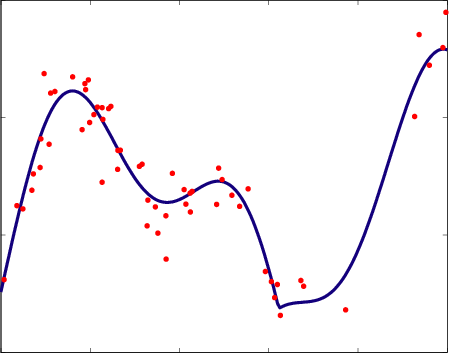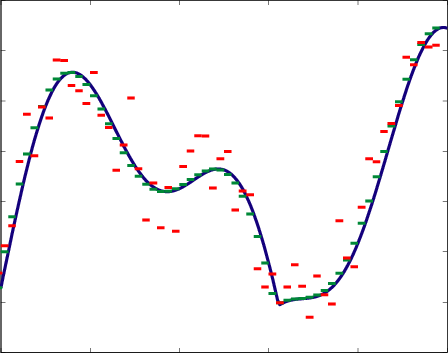# 0.4 An example of the use of sieves for complexity regularization

 Page 1 / 1

Consider the following setting. Let

$Y={f}^{*}\left(X\right)+W,$

where X is a random variable (r.v.) on $\mathcal{X}=\left[0,1\right]$ , $W$ is a r.v. on $\mathcal{Y}=\mathbf{R},$ independent of $X$ and satisfying

$E\left[W\right]=0\phantom{\rule{1.em}{0ex}}\text{and}\phantom{\rule{1.em}{0ex}}E\left[{W}^{2}\right]={\sigma }^{2}<\infty .$

Finally let ${f}^{*}:\left[0,1\right]\to \mathbf{R}$ be a function satisfying

$|{f}^{*}\left(t\right)-{f}^{*}\left(s\right)|\le L|t-s|,\phantom{\rule{4pt}{0ex}}\forall t,s\in \left[0,1\right],$

where $L>0$ is a constant. A function satisfying condition [link] is said to be Lipschitz on $\left[0,1\right]$ . Notice that such a function must be continuous, but it is not necessarilydifferentiable. An example of such a function is depicted in [link] (a).Example of a Lipschitz function, and our observations setting. (a) random sampling of f * , the points correspond to ( X i , Y i ) , i = 1 , ... , n ; (b) deterministic sampling of f * , the points correspond to ( i / n , Y i ) , i = 1 , ... , n .

Note that

$\begin{array}{ccc}\hfill E\left[Y|X=x\right]& =& E\left[{f}^{*}\left(X\right)+W|X=x\right]\hfill \\ & =& E\left[{f}^{*}\left(x\right)+W|X=x\right]\hfill \\ & =& {f}^{*}\left(x\right)+E\left[W\right]={f}^{*}\left(x\right).\hfill \end{array}$

Consider our usual setup: Estimate ${f}^{*}$ using $n$ training examples

$\begin{array}{c}\hfill {\left\{{X}_{i},{Y}_{i}\right\}}_{i=1}^{n}\stackrel{i.i.d.}{\sim }{P}_{XY},\\ \hfill {Y}_{i}={f}^{*}\left({X}_{i}\right)+{W}_{i},\phantom{\rule{4pt}{0ex}}i=\left\{1,...,n\right\},\end{array}$

where $\stackrel{i.i.d.}{\sim }$ means independently and identically distributed . [link] (a) illustrates this setup.

In many applications we can sample $\mathcal{X}=\left[0,1\right]$ as we like, and not necessarily at random. For example we can take $n$ samples uniformly on [0,1]

$\begin{array}{ccc}\hfill {x}_{i}& =& \frac{i}{n},\phantom{\rule{4pt}{0ex}}i=1,...,n\phantom{\rule{0.277778em}{0ex}},\hfill \\ \hfill {Y}_{i}& =& f\left({x}_{i}\right)+{W}_{i}\hfill \\ & =& f\left(\frac{i}{n}\right)+{W}_{i}.\hfill \end{array}$

We will proceed with this setup (as in [link] (b)) in the rest of the lecture.

Our goal is to find ${\stackrel{^}{f}}_{n}$ such that $E\left[\parallel {f}^{*}-{\stackrel{^}{f}}_{n}{\parallel }^{2}\right]\to 0,\phantom{\rule{4.pt}{0ex}}\text{as}\phantom{\rule{4.pt}{0ex}}n\to 0$ (here $\parallel ·\parallel$ is the usual ${L}_{2}$ -norm; i.e., $\parallel {f}^{*}-{\stackrel{^}{f}}_{n}{\parallel }^{2}={\int }_{0}^{1}{|{f}^{*}\left(t\right)-{\stackrel{^}{f}}_{n}\left(t\right)|}^{2}dt$ ).

Let

$\mathcal{F}=\left\{f:\phantom{\rule{4.pt}{0ex}}f\phantom{\rule{4.pt}{0ex}}\text{is}\phantom{\rule{4.pt}{0ex}}\text{Lipschitz}\phantom{\rule{4.pt}{0ex}}\text{with}\phantom{\rule{4.pt}{0ex}}\text{constant}\phantom{\rule{4.pt}{0ex}}L\right\}.$

The Riskis defined as

$R\left(f\right)=\parallel {f}^{*}{-f\parallel }^{2}={\int }_{0}^{1}{|{f}^{*}\left(t\right)-f\left(t\right)|}^{2}dt.$

The Expected Risk (recall that ourestimator ${\stackrel{^}{f}}_{n}$ is based on $\left\{{x}_{i},{Y}_{i}\right\}$ and hence is a r.v.) is defined as

$E\left[R\left({\stackrel{^}{f}}_{n}\right)\right]=E\left[\parallel {f}^{*}-{\stackrel{^}{f}}_{n}{\parallel }^{2}\right].$

Finally the Empirical Riskis defined as

${\stackrel{^}{R}}_{n}\left(f\right)=\frac{1}{n}\sum _{i=1}^{n}{\left(f,\left(\frac{i}{n}\right),-,{Y}_{i}\right)}^{2}.$

Let $0<{m}_{1}\le {m}_{2}\le {m}_{3}\le \cdots$ be a sequence of integers satisfying ${m}_{n}\to \infty$ as $n\to \infty$ , and ${k}_{n}{m}_{n}=n$ for some integer ${k}_{n}>0$ . That is, for each value of $n$ there is an associated integer value ${m}_{n}$ . Define the Sieve ${\mathcal{F}}_{1},\phantom{\rule{0.277778em}{0ex}}{\mathcal{F}}_{2},\phantom{\rule{0.277778em}{0ex}}{\mathcal{F}}_{3},\phantom{\rule{0.277778em}{0ex}}...,$

${\mathcal{F}}_{n}=\left\{f,:,f,\left(t\right),=,\sum _{j=1}^{{m}_{n}},{c}_{j},\phantom{\rule{0.166667em}{0ex}},{\mathbf{1}}_{\left\{\frac{j-1}{{m}_{n}}\le t<\frac{j}{{m}_{n}}\right\}},,,\phantom{\rule{4pt}{0ex}},{c}_{j},\in ,\mathbf{R}\right\}.$

${\mathcal{F}}_{n}$ is the space of functions that are constant on intervals

${\text{I}}_{j,{m}_{n}}\equiv \left[\frac{j-1}{{m}_{n}},,,\frac{j}{{m}_{n}}\right),\phantom{\rule{4pt}{0ex}}j=1,...,{m}_{n}.$

From here on we will use $m$ and $k$ instead of ${m}_{n}$ and ${k}_{n}$ (dropping the subscript $n$ ) for notational ease. Define

${f}_{n}\left(t\right)=\sum _{j=1}^{m}{c}_{j}^{*}\phantom{\rule{0.166667em}{0ex}}{\mathbf{1}}_{\left\{t\in {I}_{j,m}\right\}},\phantom{\rule{4.pt}{0ex}}\phantom{\rule{4.pt}{0ex}}\phantom{\rule{4.pt}{0ex}}\text{where}\phantom{\rule{4.pt}{0ex}}\phantom{\rule{4.pt}{0ex}}\phantom{\rule{4.pt}{0ex}}{c}_{j}^{*}=\frac{1}{k}\sum _{i:\frac{i}{n}\in {I}_{j,m}}{f}^{*}\left(\frac{i}{n}\right).$

Note that ${f}_{n}\in {\mathcal{F}}_{n}$ .

## Exercise 1

Upper bound $\parallel {f}^{*}-{f}_{n}{\parallel }^{2}$ .

$\begin{array}{ccc}\hfill \parallel {f}^{*}{-f\parallel }^{2}& =& {\int }_{0}^{1}{|{f}^{*}\left(t\right)-{f}_{n}\left(t\right)|}^{2}dt\hfill \\ & =& \sum _{j=1}^{m}{\int }_{{I}_{j,m}}{|{f}^{*}\left(t\right)-{f}_{n}\left(t\right)|}^{2}dt\hfill \\ & =& \sum _{j=1}^{m}{\int }_{{I}_{j,m}}{|{f}^{*}\left(t\right)-{c}_{j}^{*}|}^{2}dt\hfill \\ & =& \sum _{j=1}^{m}{\int }_{{I}_{j,m}}{\left|{f}^{*},\left(t\right),-,\frac{1}{k},\sum _{i:\frac{i}{n}\in {I}_{j,m}},{f}^{*},\left(\frac{i}{n}\right)\right|}^{2}dt\hfill \\ & =& \sum _{j=1}^{m}{\int }_{{I}_{j,m}}{\left(\frac{1}{k},\left|\sum _{i:\frac{i}{n}\in {I}_{j,m}},\left({f}^{*},\left(t\right),-,{f}^{*},\left(\frac{i}{n}\right)\right)\right|\right)}^{2}dt\hfill \\ & \le & \sum _{j=1}^{m}{\int }_{{I}_{j,m}}{\left(\frac{1}{k},\sum _{i:\frac{i}{n}\in {I}_{j,m}},\left|{f}^{*},\left(t\right),-,{f}^{*},\left(\frac{i}{n}\right)\right|\right)}^{2}dt\hfill \\ & \le & \sum _{j=1}^{m}{\int }_{{I}_{j,m}}{\left(\frac{1}{k},\sum _{i:\frac{i}{n}\in {I}_{j,m}},\frac{L}{m}\right)}^{2}dt\hfill \\ & =& \sum _{j=1}^{m}{\int }_{{I}_{j,m}}{\left(\frac{L}{m}\right)}^{2}dt\hfill \\ & =& \sum _{j=1}^{m}\frac{1}{m}{\left(\frac{L}{m}\right)}^{2}={\left(\frac{L}{m}\right)}^{2}.\hfill \end{array}$

The above implies that $\parallel {f}^{*}-{f}_{n}{\parallel }^{2}\to 0\phantom{\rule{4.pt}{0ex}}\text{as}\phantom{\rule{4.pt}{0ex}}n\to \infty ,$ since $m={m}_{n}\to \infty \phantom{\rule{4.pt}{0ex}}\text{as}\phantom{\rule{4.pt}{0ex}}n\to \infty$ . In words, with $n$ sufficiently large we can approximate ${f}^{*}$ to arbitrary accuracy using models in ${\mathcal{F}}_{n}$ (even if the functions we are using to approximate ${f}^{*}$ are not Lipschitz!).

For any $f\in {\mathcal{F}}_{n},$ $f={\sum }_{j=1}^{m}{c}_{j}\phantom{\rule{0.166667em}{0ex}}{\mathbf{1}}_{\left\{t\in {I}_{j,m}\right\}},$ we have

${\stackrel{^}{R}}_{n}\left(f\right)=\frac{1}{n}\sum _{j=1}^{m}\left(\sum _{i:\frac{i}{n}\in {I}_{j,m}},{\left({c}_{j}-{Y}_{i}\right)}^{2}\right).$

Let ${\stackrel{^}{f}}_{n}=arg{min}_{f\in {\mathcal{F}}_{n}}{\stackrel{^}{R}}_{n}\left(f\right).$ Then

${\stackrel{^}{f}}_{n}\left(t\right)=\sum _{j=1}^{m}{\stackrel{^}{c}}_{j}\phantom{\rule{0.166667em}{0ex}}{\mathbf{1}}_{\left\{t\in {I}_{j,m}\right\}},\phantom{\rule{4.pt}{0ex}}\phantom{\rule{4.pt}{0ex}}\phantom{\rule{4.pt}{0ex}}\text{where}\phantom{\rule{4.pt}{0ex}}\phantom{\rule{4.pt}{0ex}}\phantom{\rule{4.pt}{0ex}}{\stackrel{^}{c}}_{j}=\frac{1}{k}\sum _{i:\frac{i}{n}\in {I}_{j,m}}{Y}_{i}$

## Exercise 2

Note that $E\left[{\stackrel{^}{c}}_{j}\right]={c}_{j}^{*}$ and therefore $E\left[{\stackrel{^}{f}}_{n}\left(t\right)\right]={f}_{n}\left(t\right)$ . Lets analyze now the expected risk of ${\stackrel{^}{f}}_{n}$ :

$\begin{array}{ccc}\hfill E\left[\parallel {f}^{*}-{\stackrel{^}{f}}_{n}{\parallel }^{2}\right]& =& E\left[\parallel {f}^{*}-{f}_{n}+{f}_{n}-{\stackrel{^}{f}}_{n}{\parallel }^{2}\right]\hfill \\ & =& \parallel {f}^{*}-{f}_{n}{\parallel }^{2}+E\left[\parallel {f}_{n}-{\stackrel{^}{f}}_{n}{\parallel }^{2}\right]+2E\left[⟨{f}^{*}-{f}_{n},{f}_{n}-{\stackrel{^}{f}}_{n}⟩\right]\hfill \\ & =& \parallel {f}^{*}-{f}_{n}{\parallel }^{2}+E\left[\parallel {f}_{n}-{\stackrel{^}{f}}_{n}{\parallel }^{2}\right]+2⟨{f}^{*}-{f}_{n},E\left[{f}_{n}-{\stackrel{^}{f}}_{n}\right]⟩\hfill \\ & =& \parallel {f}^{*}-{f}_{n}{\parallel }^{2}+E\left[\parallel {f}_{n}-{\stackrel{^}{f}}_{n}{\parallel }^{2}\right],\hfill \end{array}$

where the final step follows from the fact that $E\left[{\stackrel{^}{f}}_{n}\left(t\right)\right]={f}_{n}\left(t\right)$ . A couple of important remarks pertaining the right-hand-side of equation [link] : The first term, $\parallel {f}^{*}-{f}_{n}{\parallel }^{2}$ , corresponds to the approximation error, and indicates how well can we approximate the function ${f}^{*}$ with a function from ${\mathcal{F}}_{n}$ . Clearly, the larger the class ${\mathcal{F}}_{n}$ is, the smallest we can make this term. This term is precisely the squared bias of the estimator ${\stackrel{^}{f}}_{n}$ . The second term, $E\left[\parallel {f}_{n}-{\stackrel{^}{f}}_{n}{\parallel }^{2}\right]$ , is the estimation error, the variance of our estimator. We will see that the estimation erroris small if the class of possible estimators ${\mathcal{F}}_{n}$ is also small.

The behavior of the first term in [link] was already studied. Consider the other term:

$\begin{array}{ccc}\hfill E\left[\parallel {f}_{n}-{\stackrel{^}{f}}_{n}{\parallel }^{2}\right]& =& E\left[{\int }_{0}^{1},{|{f}_{n}\left(t\right)-{\stackrel{^}{f}}_{n}\left(t\right)|}^{2},d,t\right]\hfill \\ & =& E\left[\sum _{j=1}^{m},{\int }_{{I}_{j,m}},{|{c}_{j}^{*}-{\stackrel{^}{c}}_{j}|}^{2},d,t\right]\hfill \\ & =& \sum _{j=1}^{m}{\int }_{{I}_{j,m}}E\left[|{c}_{j}^{*}-{\stackrel{^}{c}}_{j}{|}^{2}\right]\phantom{\rule{0.166667em}{0ex}}dt\hfill \\ & =& \sum _{j=1}^{m}{\int }_{{I}_{j,m}}\frac{E\left[{W}^{2}\right]}{k}\phantom{\rule{0.166667em}{0ex}}dt\hfill \\ & \le & \sum _{j=1}^{m}{\int }_{{I}_{j,m}}\frac{{\sigma }^{2}}{k}\phantom{\rule{0.166667em}{0ex}}dt\hfill \\ & =& \sum _{j=1}^{m}\frac{1}{m}\frac{{\sigma }^{2}}{k}=\frac{{\sigma }^{2}}{k}\phantom{\rule{4pt}{0ex}}=\phantom{\rule{4pt}{0ex}}\frac{m}{n}\phantom{\rule{0.166667em}{0ex}}{\sigma }^{2}\hfill \end{array}.$

Combining all the facts derived we have

$E\left[\parallel {f}^{*}-{\stackrel{^}{f}}_{n}{\parallel }^{2}\right]\phantom{\rule{4pt}{0ex}}\le \phantom{\rule{4pt}{0ex}}\frac{{L}^{2}}{{m}^{2}}\phantom{\rule{4pt}{0ex}}+\phantom{\rule{4pt}{0ex}}\frac{m}{n}\phantom{\rule{0.166667em}{0ex}}{\sigma }^{2}\phantom{\rule{4pt}{0ex}}=\phantom{\rule{4pt}{0ex}}O\left(max,\left\{\frac{1}{{m}^{2}},,,\frac{m}{n}\right\}\right).$

This equation used Big-O notation.

What is the best choice of $m$ ? If $m$ is small then the approximation error ( i.e., $O\left(1/{m}^{2}\right)$ ) is going to be large, but the estimation error ( i.e., $O\left(m/n\right)$ ) is going to be small, and vice-versa. This two conflicting goals provide a tradeoff that directs our choice of $m$ (as a function of $n$ ). In [link] we depict this tradeoff. In [link] (a) we considered a large ${m}_{n}$ value, and we see that the approximation of ${f}^{*}$ by a function in the class ${\mathcal{F}}_{n}$ can be very accurate (that is, our estimate will have a small bias), but when we use the measured dataour estimate looks very bad (high variance). On the other hand, as illustrated in [link] (b), using a very small ${m}_{n}$ allows our estimator to get very close to the best approximating function in the class ${\mathcal{F}}_{n}$ , so we have a low variance estimator, but the bias of our estimator ( i.e., the difference between ${f}_{n}$ and ${f}^{*}$ ) is quite considerable.Approximation and estimation of f * (in blue) for n = 60 . The function f n is depicted in green and the function f ^ n is depicted in red. In (a)we have m = 60 and in (b) we have m = 6 .

We need to balance the two terms in the right-hand-side of [link] in order to maximize the rate of decay (with $n$ ) of the expected risk. This implies that $\frac{1}{{m}^{2}}=\frac{m}{n}$ therefore ${m}_{n}={n}^{1/3}$ and the Mean Squared Error (MSE) is

$E\left[\parallel {f}_{n}-{\stackrel{^}{f}}_{n}{\parallel }^{2}\right]=O\left({n}^{-2/3}\right).$

So the sieve ${\mathcal{F}}_{1},\phantom{\rule{0.277778em}{0ex}}{\mathcal{F}}_{2},\phantom{\rule{0.277778em}{0ex}}\cdots$ with

${\mathcal{F}}_{n}=\left\{f,:,f,\left(t\right),=,\sum _{j=1}^{{m}_{n}},{c}_{j},\phantom{\rule{0.166667em}{0ex}},{\mathbf{1}}_{\left\{\frac{j-1}{{m}_{n}}\le t<\frac{j}{{m}_{n}}\right\}},,,\phantom{\rule{4pt}{0ex}},{c}_{j},\in ,\mathbf{R}\right\},$

produces a $\mathcal{F}$ -consistent estimator for ${f}^{*}=E\left[Y|X+x\right]\in \mathcal{F}$ .

It is interesting to note that the rate of decay of the MSE we obtainwith this strategy cannot be further improved by using more sophisticated estimation techniques (that is, ${n}^{-2/3}$ is the minimax MSE rate for this problem). Also, rather surprisingly, we are considering classes of models ${\mathcal{F}}_{n}$ that are actually not Lipschitz, therefore our estimator of ${f}^{*}$ is not a Lipschitz function, unlike ${f}^{*}$ itself.

how can chip be made from sand
are nano particles real
yeah
Joseph
Hello, if I study Physics teacher in bachelor, can I study Nanotechnology in master?
no can't
Lohitha
where we get a research paper on Nano chemistry....?
nanopartical of organic/inorganic / physical chemistry , pdf / thesis / review
Ali
what are the products of Nano chemistry?
There are lots of products of nano chemistry... Like nano coatings.....carbon fiber.. And lots of others..
learn
Even nanotechnology is pretty much all about chemistry... Its the chemistry on quantum or atomic level
learn
da
no nanotechnology is also a part of physics and maths it requires angle formulas and some pressure regarding concepts
Bhagvanji
hey
Giriraj
Preparation and Applications of Nanomaterial for Drug Delivery
revolt
da
Application of nanotechnology in medicine
has a lot of application modern world
Kamaluddeen
yes
narayan
what is variations in raman spectra for nanomaterials
ya I also want to know the raman spectra
Bhagvanji
I only see partial conversation and what's the question here!
what about nanotechnology for water purification
please someone correct me if I'm wrong but I think one can use nanoparticles, specially silver nanoparticles for water treatment.
Damian
yes that's correct
Professor
I think
Professor
Nasa has use it in the 60's, copper as water purification in the moon travel.
Alexandre
nanocopper obvius
Alexandre
what is the stm
is there industrial application of fullrenes. What is the method to prepare fullrene on large scale.?
Rafiq
industrial application...? mmm I think on the medical side as drug carrier, but you should go deeper on your research, I may be wrong
Damian
How we are making nano material?
what is a peer
What is meant by 'nano scale'?
What is STMs full form?
LITNING
scanning tunneling microscope
Sahil
how nano science is used for hydrophobicity
Santosh
Do u think that Graphene and Fullrene fiber can be used to make Air Plane body structure the lightest and strongest. Rafiq
Rafiq
what is differents between GO and RGO?
Mahi
what is simplest way to understand the applications of nano robots used to detect the cancer affected cell of human body.? How this robot is carried to required site of body cell.? what will be the carrier material and how can be detected that correct delivery of drug is done Rafiq
Rafiq
if virus is killing to make ARTIFICIAL DNA OF GRAPHENE FOR KILLED THE VIRUS .THIS IS OUR ASSUMPTION
Anam
analytical skills graphene is prepared to kill any type viruses .
Anam
Any one who tell me about Preparation and application of Nanomaterial for drug Delivery
Hafiz
what is Nano technology ?
write examples of Nano molecule?
Bob
The nanotechnology is as new science, to scale nanometric
brayan
nanotechnology is the study, desing, synthesis, manipulation and application of materials and functional systems through control of matter at nanoscale
Damian
Got questions? Join the online conversation and get instant answers!

#### Get Jobilize Job Search Mobile App in your pocket Now!By JavaChamp TeamBy Eric CrawfordBy OpenStaxBy OpenStaxBy OpenStaxBy Janet ForresterBy Edgar DelgadoBy Yacoub JayoghliBy OpenStaxBy OpenStax